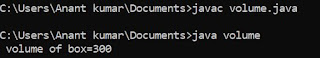## Wednesday, 4 April 2018

### Program to calculate the area of the circle in C

This is program for calculating the area of the circle in C.Here firstly we have to declare the two variable and then we have to input the values of radius of the circles and there is having use for the formula for area.Now we are going to write the program.

#include<stdio.h>
void main()
{
float area,r;
clrscr ();
printf ("\n Enter the values of r:);
scanf (%f ",& r);
area= 3.14*r*r;
printf ("\n area =%f'",area);
getch();
}